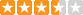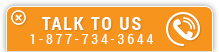# Carnot Cycle Analysis

Course Number: M-1030
Credit: 1 PDH
Subject Matter Expert: Gordan Feric, P.E.
Price: \$29.95 Purchase using Reward Tokens. Details9 reviews
Overview

#### In Carnot Cycle Analysis, you'll learn ...

• Understand basic energy conversion engineering assumptions and equations
• Know basic components of the Carnot Cycle and its T - s diagram
• Be familiar with the Carnot Cycle operation
• Understand general Carnot Cycle performance trends

#### OverviewPreview a portion of this course before purchasing it.

Credit: 1 PDH

Length: 10 pages

The Carnot Cycle is an ideal simple cycle consisting of isentropic compression and expansion and isothermal heat addition and heat rejection. The Carnot Cycle thermal efficiency determines the highest thermal efficiency that a heat engine can achieve. In this one hour online course, the closed, simple Carnot Cycle used for stationary power generation is considered.

The Carnot Cycle thermal efficiency is presented only for the air as the working fluid. The thermal efficiency derivation is presented with a simple mathematical approach. The Carnot Cycle is presented in a T - s diagram and its major performance trends are plotted in a few figures as a function of heat addition and heat rejection temperature values.

In this course, the student gets familiar with the Carnot Cycle, its components, T - s diagram, operation and major performance trends.

#### Specific Knowledge or Skill Obtained

This course teaches the following specific knowledge and skills:

• Understand basic energy conversion engineering assumptions and equations
• Know basic components of the Carnot Cycle and its T - s diagram
• Be familiar with the Carnot Cycle operation
• Understand general Carnot Cycle performance trends

#### Certificate of Completion

You will be able to immediately print a certificate of completion after passing a multiple-choice quiz consisting of 10 questions. PDH credits are not awarded until the course is completed and quiz is passed.

Board Acceptance
 This course is applicable to professional engineers in: Alabama (P.E.) Alaska (P.E.) Arkansas (P.E.) Delaware (P.E.) Florida (P.E. Area of Practice) Georgia (P.E.) Idaho (P.E.) Illinois (P.E.) Illinois (S.E.) Indiana (P.E.) Iowa (P.E.) Kansas (P.E.) Kentucky (P.E.) Louisiana (P.E.) Maine (P.E.) Maryland (P.E.) Michigan (P.E.) Minnesota (P.E.) Mississippi (P.E.) Missouri (P.E.) Montana (P.E.) Nebraska (P.E.) Nevada (P.E.) New Hampshire (P.E.) New Jersey (P.E.) New Mexico (P.E.) New York (P.E.) North Carolina (P.E.) North Dakota (P.E.) Ohio (P.E. Self-Paced) Oklahoma (P.E.) Oregon (P.E.) Pennsylvania (P.E.) South Carolina (P.E.) South Dakota (P.E.) Tennessee (P.E.) Texas (P.E.) Utah (P.E.) Vermont (P.E.) Virginia (P.E.) West Virginia (P.E.) Wisconsin (P.E.) Wyoming (P.E.)
Reviews (9)
More DetailsPreview a portion of this course before purchasing it.

Credit: 1 PDH

Length: 10 pages